# nowhere-zero flow, edge-colorings, regular graphs

## Circular flow number of regular class 1 graphs ★★

Author(s): Steffen

A nowhere-zero-flowonis an orientation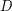oftogether with a functionfrom the edge set ofinto the real numbers such that, for all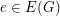, and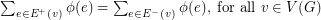. The circular flow number ofis inf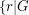has a nowhere-zero-flow, and it is denoted by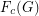.

A graph with maximum vertex degreeis a class 1 graph if its edge chromatic number is.

Conjecture   Letbe an integer anda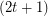-regular graph. Ifis a class 1 graph, then.